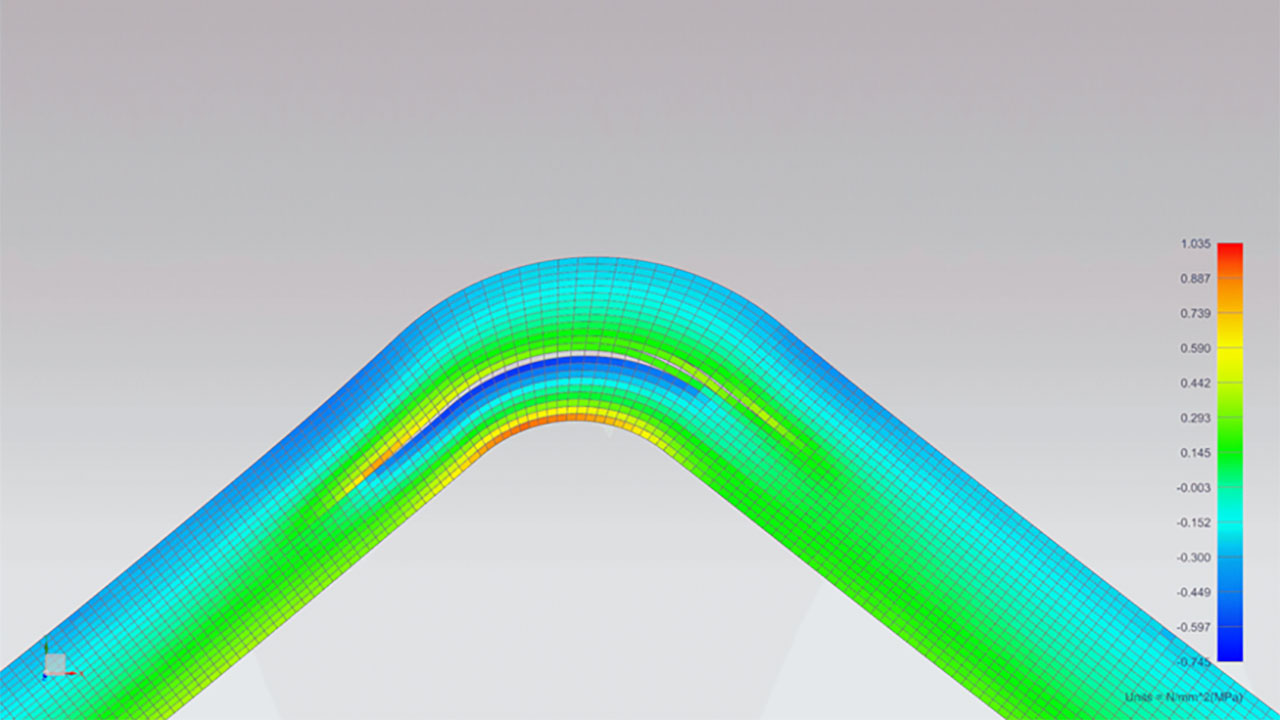# Understand the basics and mathematics of non-linear finite element analysis

On-Demand Webinar | 25 minutes

Attend this webinar and learn the basics of non-linear finite element analysis and how non-linear FE analysis allows you to simulate complex phenomena like plasticity, collapse, non linear bucklingUnderstanding the physical properties and fundamental behavior of a non-linear structure is essential in order to design a competitive product or troubleshoot unexpected issues. Non-linear finite element analysis is the tool allowing to take into account material and geometric non-linarities in order to simulate complex phenomena like plasticity, collapse, non- linear buckling

In this first webinar (basics, part 1), you will learn:

• What makes a structure non-linear
• The principles of non-linear FE simulation
• Typical examples of non-linear FE models

The second webinar (advanced non-linear FE analysis) gives you the insights in :

• Numerical aspects of non-linear FE analysis
• How to address shell and beams, composites, rotor dynamics, …
• Coupled problems like thermos-mechanical analysis, fluid structure interaction